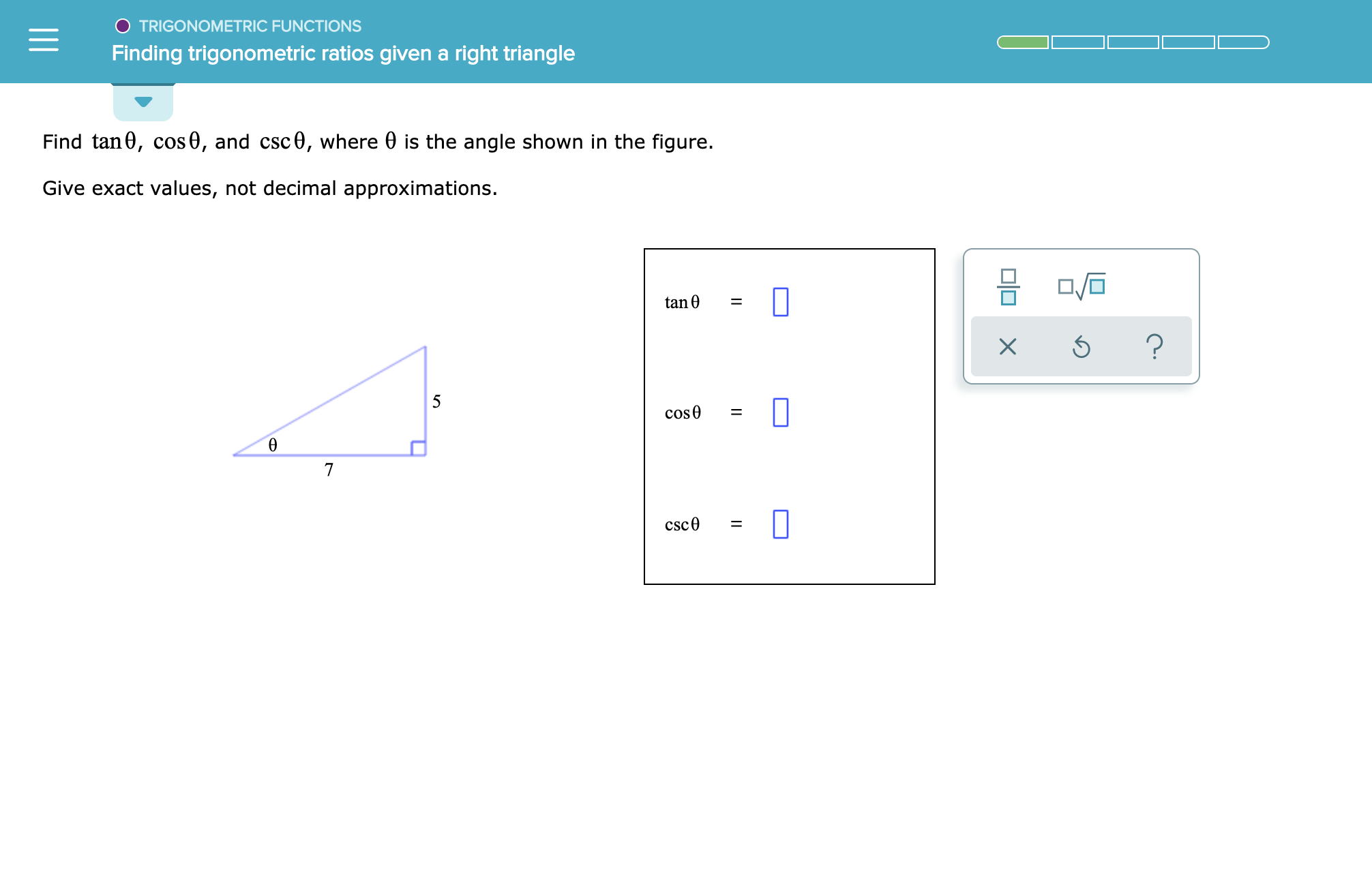# TRIGONOMETRIC FUNCTIONSFinding trigonometric ratios given a right triangleFind tan0, cos0, and csc0, where 0 is the angle shown in the figure.Give exact values, not decimal approximations.tan 0=?X5cose7csc0

Question
15 views

See attachmenthelp_outlineImage TranscriptioncloseTRIGONOMETRIC FUNCTIONS Finding trigonometric ratios given a right triangle Find tan0, cos0, and csc0, where 0 is the angle shown in the figure. Give exact values, not decimal approximations. tan 0 = ? X 5 cose 7 csc0 fullscreen
check_circle

Step 1

Given: -

Step 2

To find: -

Step 3

Calculation...

### Want to see the full answer?

See Solution

#### Want to see this answer and more?

Solutions are written by subject experts who are available 24/7. Questions are typically answered within 1 hour.*

See Solution
*Response times may vary by subject and question.
Tagged in

### Other